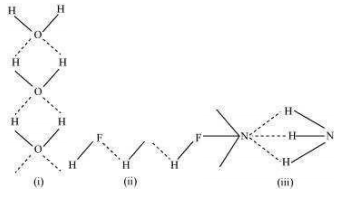9.14 Among ${\mathrm{NH}}_{3}$, H2O and HF, which would you expect to have highest magnitude of hydrogen bonding and why?

The extent of hydrogen bonding depends upon electronegativity and the number of hydrogen atoms available for bonding. Among nitrogen, fluorine, and oxygen, the increasing order of their electronegativities are N < O < F.
Hence, the expected order of the extent of hydrogen bonding is HF > ${H}_{2}O$ > $N{H}_{3}$.
But, the actual order is ${H}_{2}O$ > HF > $N{H}_{3}$.

Although fluorine is more electronegative than oxygen, the extent of hydrogen bonding is higher in water. There is a shortage of hydrogens in HF, whereas there are exactly the right numbers of hydrogens in water. As a result, only straight chain bonding takes place. On the other hand, oxygen forms a huge ring-like structure through its high ability of hydrogen bonding.

In case of ammonia, the extent of hydrogen bonding is limited because nitrogen has only one lone pair. Therefore, it cannot satisfy all hydrogens.